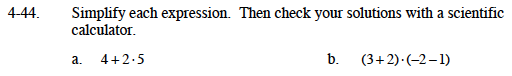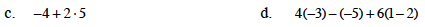### Home > MC1 > Chapter 4 > Lesson 4.1.4 > Problem4-44

4-44.
1. . Simplify each expression. Then check your solutions with a scientific calculator. Homework Help ✎

1. 4 + 2 · 5

2. (3 + 2) · ( −2 −1)

3. − 4 + 2 · 5

4. 4( −3) − ( −5) + 6(1 − 2)Refer to problem MC1 3-144 and problem MC1 3-150 if you need a reminder of how to simplify expressions.

Complete any multiplication or division.

4 + 10

4 + 10 = 14

14

Follow the correct order of operations while simplifying this expression.

−15This problem is very similar to part (a). Follow those same steps while simplifying this expression.

This problem is similar to the previous three. Be sure to simplify inside the parentheses first before adding the terms.